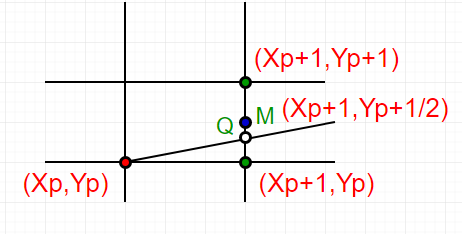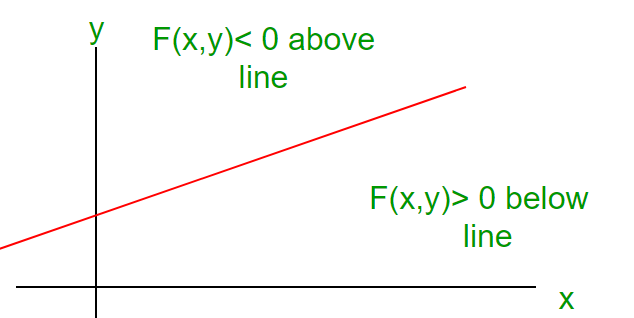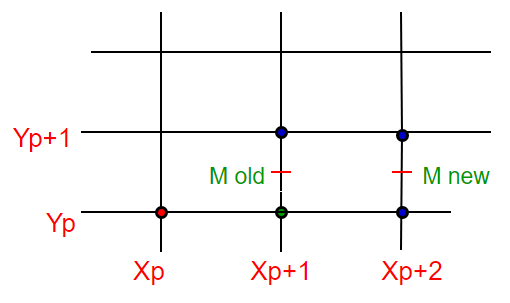# Mid-Point Line Generation Algorithm

Given coordinate of two points A(x1, y1) and B(x2, y2) such that x1 < x2 and y1 < y2. The task to find all the intermediate points required for drawing line AB on the computer screen of pixels. Note that every pixel has integer coordinates.

We have discussed below algorithms for this task.

In this post, Mid-Point Line drawing algorithm is discussed which is a different way to represent Bresenham’s algorithm introduced in previous post.

As discussed in previous post, for any given/calculated previous pixel P(Xp,Yp), there are two candidates for the next pixel closest to the line, E(Xp+1, Yp) and NE(Xp+1, Yp+1) (E stands for East and NE stands for North-East).

In Mid-Point algorithm we do following.

1. Find middle of two possible next points. Middle of E(Xp+1, Yp) and NE(Xp+1, Yp+1) is M(Xp+1, Yp+1/2).
2. If M is above the line, then choose E as next point.
3. If M is below the line, then choose NE as next point.How to find if a point is above a line or below a line?
Below are some assumptions to keep algorithm simple.

1. We draw line from left to right.
2. x1 < x2 and y1< y2
3. Slope of the line is between 0 and 1. We draw a line from lower left to upper right.

Cases other than above assumptions can be handled using reflection.

```Let us consider a line y = mx + B.
We can re-write the equation as :
y = (dy/dx)x + B or
(dy)x + B(dx) - y(dx) = 0
Let F(x, y) = (dy)x - y(dx) + B(dx)   -----(1)
Let we are given two end points of a line (under
above assumptions)
-> For all points (x,y) on the line,
the solution to F(x, y) is 0.
-> For all points (x,y) above the line,
F(x, y) result in a negative number.
-> And for all points (x,y) below the line,
F(x, y) result in a positive number.```

This relationship is used to determine the relative
position of M
M = (Xp+1, Yp+1/2)

So our decision parameter d is,
d = F(M) = F(Xp+1, Yp+1/2)

How to efficiently find new value of d from its old value?
For simplicity, let as write F(x, y) as ax + by + c.
Where a = dy
b = -dx
c = B*dx
We got these values from above equation (1)

Case 1: If E is chosen then for next point :
dnew = F(Xp+2, Yp+1/2)
= a(Xp+2) + b(Yp+1/2) + c
dold = a(Xp+1) + b(Yp+1/2) + c

Difference (Or delta) of two distances:
DELd = dnew – dold
= a(Xp+2)- a(Xp+1)+ b(Yp+1/2)- b(Yp+1/2)+ c-c
= a(Xp) +2a – a(Xp) – a
= a.
Therefore, dnew = dold + dy. (as a = dy)Case 2: If NE is chosen then for next point :
dnew = F(Xp+2, Yp+3/2)
= a(Xp+2) + b(Yp+3/2) + c
dold = a(Xp+1) + b(Yp+1/2) + c

Difference (Or delta) of two distances:
DELd = dnew -dold
= a(Xp+2)- a(Xp+1)+ b(Yp+3/2)- b(Yp+1/2)+ c-c
= a(Xp) + 2a – a(Xp) – a + b(Yp) + 3/2b – b(Yp) -1/2b
= a + b
Therefore, dnew = dold + dy – dx. (as a = dy , b = -dx)

Calculation For initial value of decision parameter d0:
d0 = F(X1+1 , Y1+1/2)
= a(X1 + 1) + b(Y1 + 1/2) +c
= aX1+ bY1 + c + a + b/2
= F(X1,Y1) + a + b/2
= a + b/2 (as F(X1, Y1) = 0 )
d0 = dy – dx/2. (as a = dy, b = -dx)

Algorithm:

```Input (X1,Y1) and (X2,Y2)
dy = Y2- Y1
dx = X2 - X1
// initial value of
// decision parameter d
d = dy - (dx/2)
x = X1 , y = Y1

// plot initial given point
Plot(x , y)

// iterate through value of X
while(x < X2)
x = x+1

// 'E' is chosen
if (d < 0)
d = d + dy

// 'NE' is chosen
else
d = d + dy - dx
y = y+1
Plot(x,y)
```

Below is the implementation of above idea.

## C++

 `// C++ program for Mid-point line generation ` `#include ` `using` `namespace` `std; ` ` `  `// Header file for including graphics functions ` `// #include ` ` `  `// midPoint function for line generation ` `void` `midPoint(``int` `X1, ``int` `Y1, ``int` `X2, ``int` `Y2) ` `{ ` `    ``// calculate dx & dy ` `    ``int` `dx = X2 - X1; ` `    ``int` `dy = Y2 - Y1; ` ` `  `    ``// initial value of decision parameter d ` `    ``int` `d = dy - (dx/2); ` `    ``int` `x = X1, y = Y1; ` ` `  `    ``// Plot initial given point ` `    ``// putpixel(x,y) can be used to print pixel ` `    ``// of line in graphics ` `    ``cout << x << ``","` `<< y << ``"\n"``; ` ` `  `    ``// iterate through value of X ` `    ``while` `(x < X2) ` `    ``{ ` `        ``x++; ` ` `  `        ``// E or East is chosen ` `        ``if` `(d < 0) ` `            ``d = d + dy; ` ` `  `        ``// NE or North East is chosen ` `        ``else` `        ``{ ` `            ``d += (dy - dx); ` `            ``y++; ` `        ``} ` ` `  `        ``// Plot intermediate points ` `        ``// putpixel(x,y) is used to print pixel ` `        ``// of line in graphics ` `        ``cout << x << ``","` `<< y << ``"\n"``; ` `    ``} ` `} ` ` `  `// Driver program ` `int` `main() ` `{ ` `    ``// graphics driver and mode ` `    ``// used in graphics.h ` `    ``// int gd = DETECT, gm; ` ` `  `    ``// Initialize graphics function ` `    ``// initgraph (&gd, &gm, ""); ` ` `  `    ``int` `X1 = 2, Y1 = 2, X2 = 8, Y2 = 5; ` `    ``midPoint(X1, Y1, X2, Y2); ` `    ``return` `0; ` `} `

## Java

 `// Java program for Mid-point  ` `// line generation ` `class` `GFG ` `{ ` `// midPoint function for line generation ` `static` `void` `midPoint(``int` `X1, ``int` `Y1, ` `                     ``int` `X2, ``int` `Y2) ` `{ ` `    ``// calculate dx & dy ` `    ``int` `dx = X2 - X1; ` `    ``int` `dy = Y2 - Y1; ` ` `  `    ``// initial value of decision ` `    ``// parameter d ` `    ``int` `d = dy - (dx/``2``); ` `    ``int` `x = X1, y = Y1; ` ` `  `    ``// Plot initial given point ` `    ``// putpixel(x,y) can be used to ` `    ``// print pixel of line in graphics ` `    ``System.out.print(x +``","` `+ y + ``"\n"``); ` ` `  `    ``// iterate through value of X ` `    ``while` `(x < X2) ` `    ``{ ` `        ``x++; ` ` `  `        ``// E or East is chosen ` `        ``if` `(d < ``0``) ` `            ``d = d + dy; ` ` `  `        ``// NE or North East is chosen ` `        ``else` `        ``{ ` `            ``d += (dy - dx); ` `            ``y++; ` `        ``} ` ` `  `        ``// Plot intermediate points ` `        ``// putpixel(x,y) is used to print ` `        ``// pixel of line in graphics ` `        ``System.out.print(x +``","` `+ y + ``"\n"``); ` `    ``} ` `} ` ` `  `// Driver code  ` `public` `static` `void` `main (String[] args) ` `{ ` `    ``int` `X1 = ``2``, Y1 = ``2``, X2 = ``8``, Y2 = ``5``; ` `    ``midPoint(X1, Y1, X2, Y2); ` `} ` `} ` ` `  `// This code is contributed by Anant Agarwal. `

## Python 3

 `# Python3 program for Mid-point ` `# line generation  ` ` `  ` `  `# midPoint function for line generation  ` `def` `midPoint(X1,Y1,X2,Y2):  ` `    ``# calculate dx & dy  ` `    ``dx ``=` `X2 ``-` `X1  ` `    ``dy ``=` `Y2 ``-` `Y1  ` ` `  `    ``# initial value of decision parameter d  ` `    ``d ``=` `dy ``-` `(dx``/``2``)  ` `    ``x ``=` `X1 ` `    ``y ``=` `Y1  ` ` `  `    ``# Plot initial given point  ` `    ``# putpixel(x,y) can be used to print pixel  ` `    ``# of line in graphics  ` `    ``print``(x,``","``,y,``"\n"``) ` `    ``# iterate through value of X  ` `    ``while` `(x < X2): ` `        ``x``=``x``+``1` `        ``# E or East is chosen ` `        ``if``(d < ``0``): ` `            ``d ``=` `d ``+` `dy  ` ` `  `        ``# NE or North East is chosen  ` `        ``else``: ` `            ``d ``=` `d ``+` `(dy ``-` `dx)  ` `            ``y``=``y``+``1` `     `  ` `  `        ``# Plot intermediate points  ` `        ``# putpixel(x,y) is used to print pixel  ` `        ``# of line in graphics  ` `        ``print``(x,``","``,y,``"\n"``)  ` `     `  ` `  `# Driver program  ` ` `  `if` `__name__``=``=``'__main__'``: ` `    ``X1 ``=` `2` `    ``Y1 ``=` `2` `    ``X2 ``=` `8` `    ``Y2 ``=` `5` `    ``midPoint(X1, Y1, X2, Y2)  ` ` `  `# This code is contributed by ash264 `

## C#

 `// C# program for Mid-point  ` `// line generation ` `using` `System; ` ` `  `class` `GFG { ` `     `  `    ``// midPoint function for line ` `    ``// generation ` `    ``static` `void` `midPoint(``int` `X1, ``int` `Y1, ` `                         ``int` `X2, ``int` `Y2) ` `    ``{ ` `         `  `        ``// calculate dx & dy ` `        ``int` `dx = X2 - X1; ` `        ``int` `dy = Y2 - Y1; ` `     `  `        ``// initial value of decision ` `        ``// parameter d ` `        ``int` `d = dy - (dx/2); ` `        ``int` `x = X1, y = Y1; ` `     `  `        ``// Plot initial given point ` `        ``// putpixel(x,y) can be used ` `        ``// to print pixel of line in ` `        ``// graphics ` `        ``Console.Write(x + ``","` `+ y + ``"\n"``); ` `     `  `        ``// iterate through value of X ` `        ``while` `(x < X2) ` `        ``{ ` `            ``x++; ` `     `  `            ``// E or East is chosen ` `            ``if` `(d < 0) ` `                ``d = d + dy; ` `     `  `            ``// NE or North East is chosen ` `            ``else` `            ``{ ` `                ``d += (dy - dx); ` `                ``y++; ` `            ``} ` `     `  `            ``// Plot intermediate points ` `            ``// putpixel(x,y) is used to print ` `            ``// pixel of line in graphics ` `            ``Console.Write(x + ``","` `+ y + ``"\n"``); ` `        ``} ` `    ``} ` `     `  `    ``// Driver code  ` `    ``public` `static` `void` `Main () ` `    ``{ ` `        ``int` `X1 = 2, Y1 = 2, X2 = 8, Y2 = 5; ` `        ``midPoint(X1, Y1, X2, Y2); ` `    ``} ` `} ` ` `  `// This code is contributed by nitin mittal. `

## PHP

 ` `

Output:

```2,2
3,3
4,3
5,4
6,4
7,5
8,5
```

Attention reader! Don’t stop learning now. Get hold of all the important DSA concepts with the DSA Self Paced Course at a student-friendly price and become industry ready.

My Personal Notes arrow_drop_up

Article Tags :
Practice Tags :

3

Please write to us at contribute@geeksforgeeks.org to report any issue with the above content.